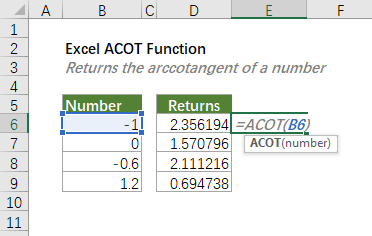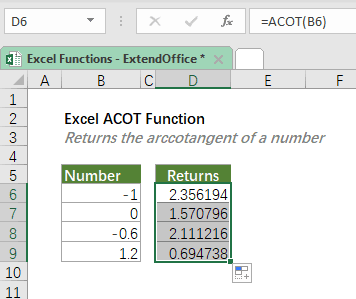## Excel ACOT Function

The ACOT function calculates the arccotangent (inverse cotangent) of a number, and returns an angle, in radians, in the range from 0 (zero) to π (pi).

Note: The ACOT function was introduced in Excel 2013. So, it is not available in earlier versions of Excel.=ACOT(number)

#### Arguments

• number (required): The value for which you want to calculate the arccotangent.

#### Return Value

The ACOT function returns a numeric value.

#### Function Notes

• The result is an angle expressed in radians. To convert the angle from radians to degrees, use the DEGREES function, or multiply the result by 180/PI().
• ACOT returns the #VALUE! error if number is not numeric.

#### Example

To calculate the arccotangent (inverse cotangent) of the numbers listed in the table as shown below, please copy or enter the below formula in the top cell (D6) of the result list, and press Enter to get the result. Then select the result cell, and drag the fill handle (the small square in the lower-right corner of the selected cell) down to apply the formula to the below cells.

=ACOT(B6)Instead of a cell reference, you can type the actual number value in the formula as shown below.

=ACOT(-1)

Note that the results are angles expressed in radians. You can nest the ACOT function into a DEGREES function to convert the angles from radians to degrees as shown below.

=DEGREES(ACOT(-1))

Alternatively, you can multiply ACOT by 180/PI().

=ACOT(-1)*180/PI()

#### Related functions

Excel ACOTH Function

The ACOTH function returns the inverse hyperbolic cotangent of a number.

Excel DEGREES Function

The DEGREES function converts an angle in radians to an angle in degrees.

Excel PI Function

The PI function returns the number 3.14159265358979 of the mathematical constant called pi.

### The Best Office Productivity Tools

#### Kutools for Excel - Helps You To Stand Out From Crowd

 Popular Features: Find, Highlight or Identify Duplicates  |  Delete Blank Rows  |  Combine Columns or Cells without Losing Data  |  Round without Formula ... Super VLookup: Multiple Criteria  |  Multiple Value  |  Across Multi-Sheets  |  Fuzzy Lookup... Adv. Drop-down List: Easy Drop Down List  |  Dependent Drop Down List  |  Multi-select Drop Down List... Column Manager: Add a Specific Number of Columns  |  Move Columns  |  Toggle Visibility Status of Hidden Columns  |  Compare Columns to Select Same & Different Cells ... Featured Features: Grid Focus  |  Design View  |  Big Formula Bar  |  Workbook & Sheet Manager | Resource Library (Auto Text)  |  Date Picker  |  Combine Worksheets  |  Encrypt/Decrypt Cells  |  Send Emails by List  |  Super Filter  |  Special Filter (filter bold/italic/strikethrough...) ... Top 15 Toolsets:  12 Text Tools (Add Text, Remove Characters ...)  |  50+ Chart Types (Gantt Chart ...)  |  40+ Practical Formulas (Calculate age based on birthday ...)  |  19 Insertion Tools (Insert QR Code, Insert Picture from Path ...)  |  12 Conversion Tools (Numbers to Words, Currency Conversion ...)  |  7 Merge & Split Tools (Advanced Combine Rows, Split Excel Cells ...)  |  ... and more

Kutools for Excel Boasts Over 300 Features, Ensuring That What You Need is Just A Click Away...#### Office Tab - Enable Tabbed Reading and Editing in Microsoft Office (include Excel)

• One second to switch between dozens of open documents!
• Reduce hundreds of mouse clicks for you every day, say goodbye to mouse hand.
• Increases your productivity by 50% when viewing and editing multiple documents.
• Brings Efficient Tabs to Office (include Excel), Just Like Chrome, Edge and Firefox.